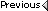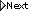» Hatrack River Forum » Active Forums » Books, Films, Food and Culture » Yo stupidly simple Calculus question

Author Topic: Yo stupidly simple Calculus question
 Blayne Bradley unregistered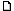posted February 07, 2010 07:20 PM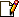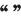okay so if I have f(x)=(x+2)^2then f'(x) = 2*(x+2)* (dx/dy) x+2 which is 1so f'(x) = 2x+4However if I want to find the integral of 2x+4so: f(x) = 2x+4 (dx)x^2+4x+CWhich I imagine if you factor it probably equals to (x+2)^2 if you solve for cx^2+2x+2x+4x^2+4x+4Which seems a little wierd, is the answer good enough at the polynomial x^2+4x+C or do I have to get it back to (x+2)^2?Next if I have (x+2)^2, do I find its integral? Foil it out and do f(x)= x^2+4x+4 (dx)?In which case it should be (1/3)(x^3) + 2x^2 + 4x + C?Or is there a reverse chain rule I can use?(1/3)[(x+2)^3](x^2+2x)+C?//one third times x+2 cubed times the intergral of the inside plus a constant? IP: Logged |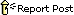Phanto Member Member # 5897posted February 07, 2010 08:03 PM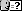Your question doesn't really make sense to me. quote:is the answer good enough at the polynomial x^2+4x+C or do I have to get it back to (x+2)^2?The integral of 2x + 4 is x^2 + 4x + C... where are you getting the whole weird (x+2)^2 stuff from?The fact that you can rewrite an equation in another way doesn't mean you should, and unless you know what C is for some reason then it is unknown. Posts: 3060 | Registered: Nov 2003  |  IP: Logged |Blayne Bradley unregisteredposted February 07, 2010 08:25 PMThe point is say I have f(x) = (x+2)^2The idea is figuring out how to integrate it and how to integrate back from its derivative. IP: Logged |fugu13 Member Member # 2859posted February 07, 2010 10:19 PM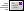You can't, perfectly. Information is lost. Taking the derivative of any constant is 0, so it is impossible to integrate and work out any constant terms that were part of the original.(x+2)^2 has a constant term -- writing it another way, as x^2 + 4x + 4, you can see that clearly. After taking the derivative, all information about that constant term is lost. It was a 4, but from the derivative you can't tell if it was a 23 or a 7 or 2.1 billion. So no, you can't get back to (x+2)^2 from knowing the derivative is 2x + 4. Because it could have been x^2 + 4x + 3.14159 instead of x^2 + 4x + 4.Now, if you have additional information, you can compute the constant factor. For instance, sometimes you are given the intercept or somesuch. Posts: 15770 | Registered: Dec 2001  |  IP: Logged |The Rabbit Member Member # 671posted February 08, 2010 09:38 AMquote:Originally posted by Blayne Bradley: okay so if I have f(x)=(x+2)^2then f'(x) = 2*(x+2)* (dx/dy) x+2 which is 1so f'(x) = 2x+4 You've lost me completely.What is y? What variable are you differentiating with respect to?what is 1?Aside from that, the answer fugu and Phanto have given you is accurate.You loose information when you take a derivative, that's why you have to add in an arbitrary constant when you integrate. In order to recover the original function by integrating a derivative, you have to have more information. Typically, you have to know the value of the initial function at some point. Posts: 12591 | Registered: Jan 2000  |  IP: Logged |The White Whale Member Member # 6594posted February 08, 2010 09:50 AMI think he means f'(x) = 2*(x+2)*d/dx(x+2), and that d/dx(x+2) = 1. Posts: 1710 | Registered: Jun 2004  |  IP: Logged |Mike Member Member # 55posted February 10, 2010 02:21 AMquote:You loose information...Speaking of which, I can't wait 'til my information bow arrives in the mail.Posts: 1810 | Registered: Jan 1999  |  IP: Logged |willthesane Member Member # 11754posted February 11, 2010 01:29 PMthe integral from a to b of f(g(x))*g'(x) is the same as the integral from g(a) to g(b) of f(x).so, the integral of f(x)=(x+2)^2 dx from 0 to 2 is the same as the integral of f(u)=u^2 from 2 to 4.http://en.wikipedia.org/wiki/Integration_by_substitutionif this is what you were looking for i wish you luck. Posts: 7 | Registered: Sep 2008  |  IP: Logged |Quick Reply Message: HTML is not enabled. UBB Code is enabled. UBB Code™ Images not permitted. Instant Graemlins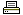Printer-friendly view of this topic Hop To: Select a Forum:   Category: Active Forums -------------------- Discussions About Orson Scott Card Books, Films, Food and Culture Uncle Orson On the Fly   Category: Archives -------------------- Landmark Threads